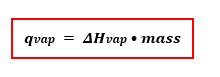# Problem: A 75.0 mL sample of water is heated to its boiling point. How much heat is required to vaporize it? (Assume a density of 1.00 g/mL.)

🤓 Based on our data, we think this question is relevant for Professor Kennedy's class at GSU.

###### FREE Expert Solution

Recall that the Heat required to vaporize or qvap can be solved using the enthalpy of vaporization of water.

The equation is:###### Problem Details
A 75.0 mL sample of water is heated to its boiling point. How much heat is required to vaporize it? (Assume a density of 1.00 g/mL.)